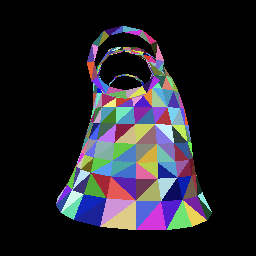# 木匣子

Web/Game/Programming/Life etc.

# 动森二维码生成器：服装类（后篇）

o model-name
v x0 y0 z0
v x1 y1 z1
v x2 y2 z2
...
vt u1 v1
vt u2 v2
vt u3 v3
...
vn nx0 ny0 nz0
vn nx1 ny1 nz1
vn nx2 ny2 nz2
...
f 1/1/1 2/2/2 3/3/3
...• 方法一：可以通过计算 XY 平面上的 AB×AP (crossProduct)，得到 Z 轴为正数则点在线段右侧（右手定则），该结果有很多含义，例如其方向为AB与AP的法向量，大小为AB与AP围成的三角形的面积的两倍。
• 方法二：计算线段AB的法向量与AP的夹角的余弦值（dotProduct)，得到正数则点在线段右侧。$$R_y(\theta) = \begin{bmatrix} cos(\theta) & 0 & sin(\theta)\\ 0 & 1 & 0\\ -sin(\theta) & 0 & cos(\theta) \end{bmatrix}$$

-- create rotation matrix on y axis
function rotateY(a)
return matrix({
{ math.cos(a), 0, math.sin(a), 0 },
{ 0, 1, 0, 0 },
{ -math.sin(a), 0, math.cos(a), 0 },
{ 0, 0, 0, 1 },
})
end


Aseprite 的插件接口比较简陋，我使用了两个按钮来控制旋转的角度，效果如下：

$$\begin{bmatrix} x_{right} & x_{up} & x_{forward} & x_{eye}\\ y_{right} & y_{up} & y_{forward} & y_{eye}\\ z_{right} & z_{up} & z_{forward} & z_{eye}\\ 0 & 0 & 0 & 1 \end{bmatrix}^{-1}$$

-- create a camera to world matrix
-- use matrix:inverse() to get the world to camera matrix
function lookAt(eye, target, up)
local globalUp = up and normalize(up) or { 0, 1, 0 }
local forward = normalize({ eye - target, eye - target, eye - target })
local right = crossProduct(globalUp, forward)
local up = crossProduct(forward, right)
return matrix({
{ right, up, forward, eye },
{ right, up, forward, eye },
{ right, up, forward, eye },
{ 0, 0, 0, 1 }
})
end


UV 映射的效果不错，接下来就是读取贴图，然后按插值手的 UV 坐标从贴图上读取颜色，绘制到三角形内：